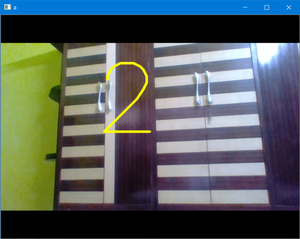Related Articles
Set Countdown timer to Capture Image using Python-OpenCV
• Last Updated : 18 Aug, 2020

Prerequisites: Introduction to OpenCV

Most of us have already captured image using countdown timer with our phones. We can do the same thing on our computer with the help of OpenCV.

But here we can specify the countdown timer instead of choosing one of the specified  countdown and whenever the particular key will be pressed (let’s say q) the countdown timer will be started and we will be displaying the countdown on our camera with the help of cv2.putText() function  and when it reaches zero we will capture the image, display the captured image for fixed number of seconds (according to our need ) and write/save the image on disk. Now let’s see how to perform this task:

Approach:

• First, we will be setting the initial value of Countdown timer in second. (We can also take this as input from user).
• Open the camera and create a video Capture object using cv2.VideoCapture().
• While camera is open
• We will read each frame and display it using cv2.imshow().
• Now we will set a key (we use q ) for the countdown to begin.
• As soon as this key will be pressed, we will start the Countdown.
• We will be keeping track of countdown with the help of time.time() function and display the countdown on the video using cv2.putText()  function.
• When it reaches zero, we will copy the current frame and write the current frame at desired location on disk by using cv2.imwrite() function.
• On pressing ‘Esc’ we will close the camera.

Below is the implementation.

## Python3

 `import` `cv2``import` `time``  ` ` ` `# SET THE COUNTDOWN TIMER``# for simplicity we set it to 3``# We can also take this as input``TIMER ``=` `int``(``20``)``  ` `# Open the camera``cap ``=` `cv2.VideoCapture(``0``)``  ` ` ` `while` `True``:``     ` `    ``# Read and display each frame``    ``ret, img ``=` `cap.read()``    ``cv2.imshow(``'a'``, img)`` ` `    ``# check for the key pressed``    ``k ``=` `cv2.waitKey(``125``)`` ` `    ``# set the key for the countdown``    ``# to begin. Here we set q``    ``# if key pressed is q``    ``if` `k ``=``=` `ord``(``'q'``):``        ``prev ``=` `time.time()`` ` `        ``while` `TIMER >``=` `0``:``            ``ret, img ``=` `cap.read()`` ` `            ``# Display countdown on each frame``            ``# specify the font and draw the``            ``# countdown using puttext``            ``font ``=` `cv2.FONT_HERSHEY_SIMPLEX``            ``cv2.putText(img, ``str``(TIMER), ``                        ``(``200``, ``250``), font,``                        ``7``, (``0``, ``255``, ``255``),``                        ``4``, cv2.LINE_AA)``            ``cv2.imshow(``'a'``, img)``            ``cv2.waitKey(``125``)`` ` `            ``# current time``            ``cur ``=` `time.time()`` ` `            ``# Update and keep track of Countdown``            ``# if time elapsed is one second ``            ``# than decrese the counter``            ``if` `cur``-``prev >``=` `1``:``                ``prev ``=` `cur``                ``TIMER ``=` `TIMER``-``1`` ` `        ``else``:``            ``ret, img ``=` `cap.read()`` ` `            ``# Display the clicked frame for 2 ``            ``# sec.You can increase time in ``            ``# waitKey also``            ``cv2.imshow(``'a'``, img)`` ` `            ``# time for which image displayed``            ``cv2.waitKey(``2000``)`` ` `            ``# Save the frame``            ``cv2.imwrite(``'camera.jpg'``, img)`` ` `            ``# HERE we can reset the Countdown timer``            ``# if we want more Capture without closing``            ``# the camera`` ` `    ``# Press Esc to exit``    ``elif` `k ``=``=` `27``:``        ``break`` ` `# close the camera``cap.release()``  ` `# close all the opened windows``cv2.destroyAllWindows()`

Output:Attention geek! Strengthen your foundations with the Python Programming Foundation Course and learn the basics.

To begin with, your interview preparations Enhance your Data Structures concepts with the Python DS Course. And to begin with your Machine Learning Journey, join the Machine Learning – Basic Level Course

My Personal Notes arrow_drop_up